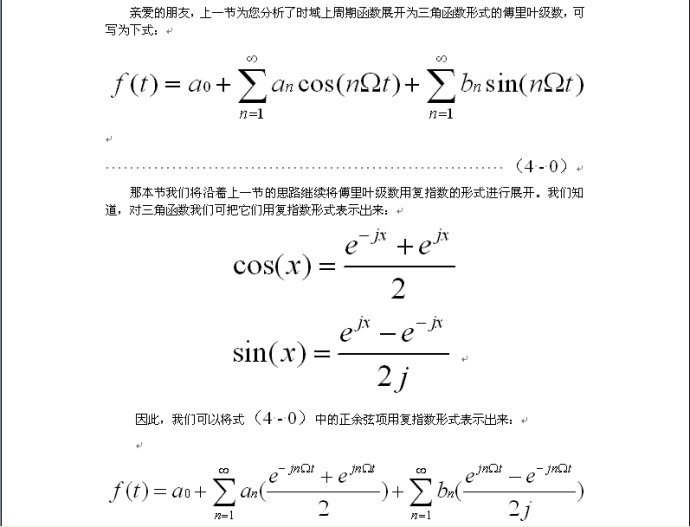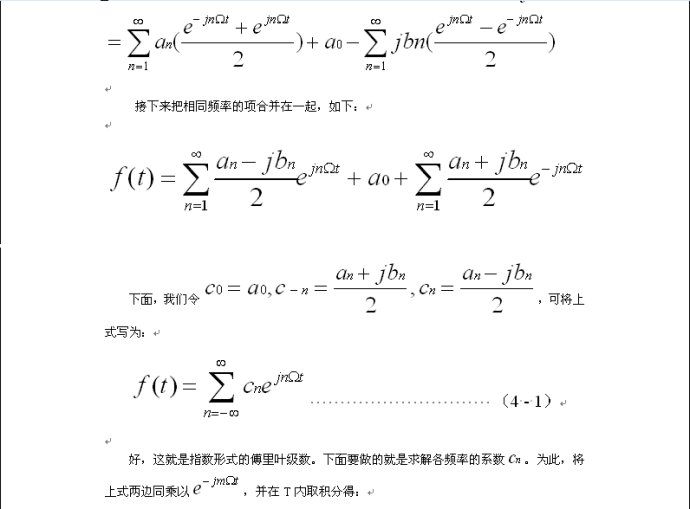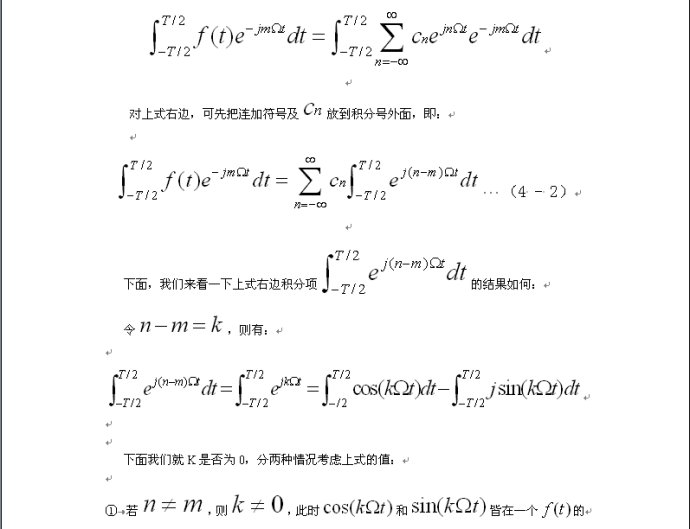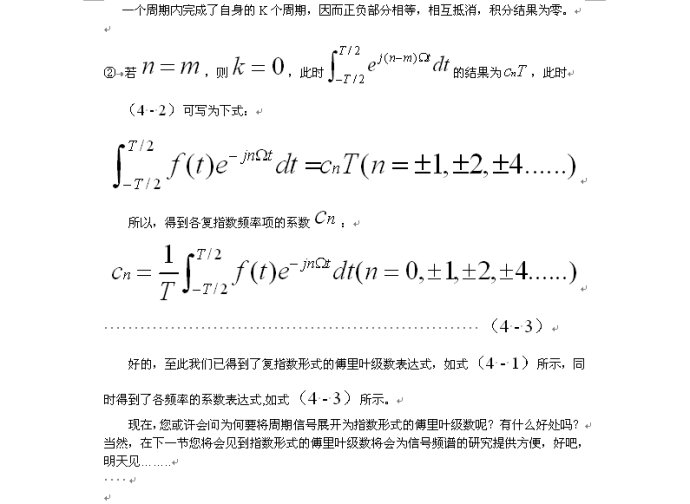• 原文：http://blog.sina.com.cn/s/blog_66585b7f0100hmhq.html  本文详细介绍了傅里叶级数的指数变换形式，将指数形式和三角形式相连起来

原文：http://blog.sina.com.cn/s/blog_66585b7f0100hmhq.html

本文详细介绍了傅里叶级数的指数变换形式，将指数形式和三角形式相连起来展开全文• 满足Direchlet条件的周期信号或特定时间区间的信号可以被傅里叶级数在功率上没有误差的表达，常见的傅里叶级数形式有两种，即： f(t)=a02+∑n=1+∞[ancos(nΩt)+bnsin(nΩt)]an=2t2−t1∫t1t2f(t)cos(nΩt)dtbn=2t...
满足Direchlet条件的周期信号或特定时间区间的信号可以被傅里叶级数在功率上没有误差的表达，常见的傅里叶级数的形式有两种，即：
\begin{aligned} f(t) &= \frac{a_0}{2}+\sum_{n=1}^{+\infty}[a_ncos(n\Omega t)+b_nsin(n\Omega t)] \\a_n&=\frac{2}{t_2-t_1}\int_{t_1}^{t_2}f(t)cos(n\Omega t)dt \\b_n&=\frac{2}{t_2-t_1}\int_{t_1}^{t_2}f(t)sin(n\Omega t)dt \end{aligned}
或
\begin{aligned} f(t)&=\frac{a_0}{2}+\sum_{n=1}^{+\infty}A_ncos(n\Omega t + \varphi_n) \\A_n &= \sqrt{a_n^2+b_n^2}, \\\varphi_n &= -arctan\frac{b_n}{a_n} \end{aligned}
这两种表达方式在物理上很容易理解，即信号可以被分解为一个直流分量，和一系列交流分量的叠加。除了这两种表达方式，傅里叶级数的复指数形式也是最常见的表达形式，这种形式在计算上具有很大的优势。
1. 如何获得傅里叶级数的复数形式？
和之前讨论的信号分解累，如果存在一组正交函数集，则信号可以通过正交函数集中的子信号的叠加进行表示。子信号的系数称为相关系数。相关内容可以回顾信号与系统（6）- 信号频域研究的思路及正交函数集。正弦函数集是一套正交函数集，除此之外，复指数函数也是正交函数集，即：
$\{ 1, e^{j\Omega t }, e^{j2\Omega t }, e^{j3\Omega t }\cdots, e^{jn\Omega t }\}$
或记为：
$\{ e^{jn\Omega t }\space|n \in I \}$
则信号$f(t)$通过复指数正交函数集展开为：
$f(t)=\sum_{n=-\infty}^{+\infty}[C_n\cdot e^{j(n\Omega t)}]$
注意求和上下限为从负无穷到正无穷，这意味着复指数形式的傅里叶变换会出现“负频率”。至于为什么出现负频率将在之后解答。
由之前正交函数集的知识可知，上式中系数$C_n$为：
$C_n=\frac{\int_{t_1}^{t_2}f(t)\cdot (e^{j{n\Omega t}})^*}{\int_{t_1}^{t_2}(e^{j{n\Omega t}})(e^{j{n\Omega t}})^*} = \frac{1}{T}\int_{t_1}^{t_2}f(t)e^{-jn\Omega t}dt$
上式即为傅里叶级数的复数形式，其中$\Omega = \frac{2\pi}{T}$，且$f(t)$是周期为T的函数，故n取不同值时的周期信号具有谐波关系（即它们都具有一个共同周期T）。n=0时对应的这一项称为直流分量，n=1时具有基波频率，称为一次谐波或基波，类似的有二次谐波，三次谐波等等。
同之前讲述的实数范围内的傅里叶级数展开一样，复指数形式的傅里叶级数展开同样具有另一种表达方式。已知傅里叶级数为：
$f(t)=\frac{a_0}{2}+\sum_{n=1}^{+\infty}A_ncos(n\Omega t + \varphi_n)$
由欧拉公式：
$cos\omega t = \frac{e^{j\omega t}+e^{-j\omega t}}{2}$
则：
\begin{aligned} f(t)&=\frac{a_0}{2}+\sum_{n=1}^{+\infty}A_ncos(n\Omega t + \varphi_n) \\&=\frac{a_0}{2}+\sum_{n=1}^{+\infty}A_n\frac{e^{j(n\Omega t + \varphi_n)}+e^{-j(n\Omega t + \varphi_n)}}{2} \\&=\frac{a_0}{2}+\sum_{n=1}^{+\infty}A_n\frac{e^{j(n\Omega t + \varphi_n)}+e^{j[-(n)\Omega t + (-\varphi_n)]}}{2} \\&=\frac{a_0}{2}+\sum_{n=1}^{+\infty}\frac{A_n}{2}e^{j(n\Omega t + \varphi_n)}+\sum_{n=-1}^{-\infty}\frac{A_n}{2}e^{j(n\Omega t + \varphi_n)} \end{aligned}
回顾傅里叶级数的第二种形式
\begin{aligned} f(t)&=\frac{a_0}{2}+\sum_{n=1}^{+\infty}A_ncos(n\Omega t + \varphi_n) \\A_n &= \sqrt{a_n^2+b_n^2}, \\\varphi_n &= -arctan\frac{b_n}{a_n} \end{aligned}
若$f(t)$是实数信号，则$A_n$是$n$的偶函数，而$\varphi_n$是$n$的奇函数。如果将求和的范围从$[1,+\infty]$扩展到$[-1,-\infty]$，则有$\varphi_{-n}=-\varphi_{n}$以及$A_{-n}=A_n$，此时$n \in[-1,-\infty]$，因此有：
\begin{aligned} f(t)&=\frac{a_0}{2}+\sum_{n=1}^{+\infty}A_ncos(n\Omega t + \varphi_n) \\&=\frac{a_0}{2}+\sum_{n=1}^{+\infty}A_n\frac{e^{j(n\Omega t + \varphi_n)}+e^{-j(n\Omega t + \varphi_n)}}{2} \\&=\frac{a_0}{2}+\sum_{n=1}^{+\infty}A_n\frac{e^{j(n\Omega t + \varphi_n)}+e^{j[-(n)\Omega t + (-\varphi_n)]}}{2} \\&=\frac{a_0}{2}+\sum_{n=1}^{+\infty}\frac{A_n}{2}e^{j(n\Omega t + \varphi_n)}+\sum_{n=-1}^{-\infty}\frac{A_n}{2}e^{j(n\Omega t + \varphi_n)} \end{aligned}
若$n=0$时$\varphi_n$记为$\varphi_0 = 0$，$A_n$记为$A_0=a_0$，则上式可以统一化表示为：
\begin{aligned} f(t)&=\frac{a_0}{2}+\sum_{n=1}^{+\infty}\frac{A_n}{2}e^{j(n\Omega t + \varphi_n)}+\sum_{n=-1}^{-\infty}\frac{A_n}{2}e^{j(n\Omega t + \varphi_n)} \\&=\frac{1}{2}\sum_{n=-\infty}^{+\infty}A_ne^{j\varphi_n}\cdot e^{jn\Omega t} \\&=\frac{1}{2}\sum_{n=-\infty}^{+\infty}\dot A_n\cdot e^{jn\Omega t} \end{aligned}
上式即傅里叶级数复数形式的第二种表达方式，其中
\begin{aligned} &\dot A_n = A_ne^{j\varphi_n} = \sqrt{a_n^2+b_n^2}(cos \varphi_n + j sin\varphi_n) \\&\varphi_n=-arctan(\frac{b_n}{a_n}) \end{aligned}
通过三角函数运算可知：
\begin{aligned} &若\space \varphi_n=-arctan(\frac{b_n}{a_n}) \\&则\space cos(\varphi_n)=\frac{a_n}{\sqrt{a_n^2+b_n^2}}, \space sin(\varphi_n)=-\frac{b_n}{\sqrt{a_n^2+b_n^2}} \end{aligned}
所以$\dot A_n$也可以表示为：
\begin{aligned} \dot A_n &= a_n - jb_n \\&=\frac{2}{T}\int_{t_1}^{t_2}f(t)cos(n\Omega t)dt-j=\frac{2}{T}\int_{t_1}^{t_2}f(t)sin(n\Omega t)dt \\&=\frac{2}{T}\int_{t_1}^{t_2}f(t)[cos(n\Omega t)-jsin(n\Omega t)] \\由e^{j\omega t}&=cos\omega t+jsin\omega t,e^{-j\omega t}=cos\omega t-jsin\omega t可知 \\&=\frac{2}{T}\int_{t_1}^{t_2}f(t)e^{-j\Omega t}dt \end{aligned}
对比第一种傅里叶级数复数形式和第二种傅里叶级数复数形式可知：
$C_n = \frac{\dot A_n}{2}=\frac{ A_ne^{j\varphi_n}}{2}=\frac{a_n - jb_n}{2}$
问题：负频率的出现有什么意义？
傅里叶级数的实数形式在物理上很好理解，但是计算相对繁琐。而复指数形式的傅里叶级数因为引入了指数运算，因此在计算上会相对容易，但是负频率的出现会让人难以理解其物理意义。观察欧拉关系或复指数的定义：
$e^{j\omega t} = cos\omega t +jsin\omega t,\space 或\space cos\omega t = \frac{e^{j\omega t}+e^{-j\omega t}}{2}$
可知，正数频率的复指数信号$e^{j\omega t}$和负数频率的复指数信号$e^{-j\omega t}$，这两者共同构成了实数信号$cos\omega t$。因此可以粗略的理解为，负频率的出现，是为了实现通过复指数信号构成一个实数信号的目的。
2. 四种傅里叶级数的形式对比
实数形式的傅里叶变换和复数形式的傅立叶变换本质上是一回事，但是表现的形式不同。他们之间存在很密切的联系，其对比如下：

傅里叶级数形式
系数或参数

$f(t) = \frac{a_0}{2}+\sum_{n=1}^{+\infty}[a_ncos(n\Omega t)+b_nsin(n\Omega t)]$
$a_n=\frac{2}{t_2-t_1}\int_{t_1}^{t_2}f(t)cos(n\Omega t)dt\\b_n=\frac{2}{t_2-t_1}\int_{t_1}^{t_2}f(t)sin(n\Omega t)dt$

$f(t)=\frac{a_0}{2}+\sum_{n=1}^{+\infty}A_ncos(n\Omega t + \varphi_n)$
$A_n = \sqrt{a_n^2+b_n^2},\\\varphi_n = -arctan\frac{b_n}{a_n}$

$f(t)=\sum_{n=-\infty}^{+\infty}[C_n\cdot e^{j(n\Omega t)}]$
$C_n=\frac{\int_{t_1}^{t_2}f(t)\cdot (e^{j{n\Omega t}})^*}{\int_{t_1}^{t_2}(e^{j{n\Omega t}})(e^{j{n\Omega t}})^*} = \frac{1}{T}\int_{t_1}^{t_2}f(t)e^{-jn\Omega t}dt\\=\frac{\dot A_n}{2}=\frac{ A_ne^{j\varphi_n}}{2}=\frac{a_n - jb_n}{2}$

$f(t)=\frac{1}{2}\sum_{n=-\infty}^{+\infty}\dot A_n\cdot e^{jn\Omega t}$
$\dot A_n = A_ne^{j\varphi_n} = \sqrt{a_n^2+b_n^2}(cos \varphi_n + j sin\varphi_n)\\= a_n - jb_n \\=\frac{2}{T}\int_{t_1}^{t_2}f(t)e^{-j\Omega t}dt$

不论哪种傅里叶级数的形式，可以看出，任何周期性的，或某特定时间区间的，满足Direchlet条件的信号都可以分解为直流分量和不同交流分量的得加。傅里叶级数的本质同样是使用子信号对复杂信号的表示这与之前时域分析中的思路是完全一致的。
当获得系统对子信号的响应，最后将子信号的响应进行叠加，即可求得系统对原信号的响应。
3. 复指数信号长什么样子？
$\{ e^{jn\Omega t }\space|n \in I \}$表示一种复正弦信号，有关这个信号的图像可以通过MATLAB或SCILAB进行绘制，如下：可以看出，复指数函数是一个螺旋线，在实数轴上是余弦曲线，在虚数轴上是正弦曲线。复指数信号中的n可以为正数也可以为负数，此时频率$n\Omega$便出现了小于零的负频率。这在物理上没有意义，但是数学上会简化运算。有关这个函数的性质以及虚数的意义，将在之后必要的时候进行讨论。
4. 函数奇偶性和傅里叶级数的关系
函数的奇偶性对傅里叶级数具有一定的影响，有时候会简化对傅里叶级数的计算

如果函数是偶函数，则其傅里叶级数中只有直流和余弦分量，即：偶函数之和仍是偶函数
如果函数是奇函数，则其傅里叶级数中只有正弦分量，即：奇函数之和仍是奇函数
符合$f(t+\frac{T}{2})=-f(t)$的函数称为奇谐函数，其傅里叶级数中仅由奇次谐波分量
符合$f(t+\frac{T}{2})=f(t)$的函数称为偶谐函数，其傅里叶级数中仅由偶次谐波分量

事实上，任何信号均可以分解成为一个奇函数和一个偶函数的和，并且信号的平移可以改变信号的奇偶性，如下所示：
$f(t)=f_e(t)+f_o(t)=\frac{f(t)+f(-t)}{2}+\frac{f(t)-f(-t)}{2}$
因此，若已知信号灯的奇偶性，则计算傅里叶系数时，仅需计算部分系数即可。
至此，傅里叶级数相关的内容完成，下一部分将阐述傅里叶级数的频谱特点。
谢谢阅读，如有不当之处，欢迎批评指正！


展开全文信号处理
• 傅里叶级数的三角函数形式，傅里叶级数复指数形式
eiθ=cosθ+isinθ⇒⎧⎩⎨⎪⎪⎪⎪cosθ=12eiθ+12e−iθsinθ=12ieiθ−12ie−iθ<!--//--><![CDATA[//><!--
e^{i\theta}=\cos\theta+i\sin\theta ⇒
\left\{
\begin{split}
& \cos\theta=\frac12e^{i\theta}+\frac12e^{-i\theta} \\
&\sin\theta=\frac1{2i}e^{i\theta}-\frac1{2i}e^{-i\theta}
\end{split}
\right.
//--><!]]>欧拉公式的存在，建立了三角函数与复指数之间的桥梁，也使得相当多的数学公式形式变得简单。

1. 傅里叶级数（fourier series）

u(t)=a02+∑+∞n=1(ancos2πnFt+bnsin2πnFt)<!--//--><![CDATA[//><!--
u(t)=\frac{a_0}2+\sum\nolimits_{n=1}^{+\infty}\left(a_n\cos2\pi nFt+b_n\sin2\pi nFt\right)
//--><!]]>2. 傅里叶级数的复指数形式

为简化运算，不妨令 θ=2πnFt<!--//--><![CDATA[//><!--
\theta=2\pi nFt
//--><!]]>，则：

u(t)=====a02+∑+∞n=1(ancos2πnFt+bnsin2πnFt)a02+∑∞n=1(ancosθ+bnsinθ)a02+∑∞n=1((12an−12ibn)eiθ+(12an+12ibn)e−iθ)a02+∑∞n=1((12an−12ibn)ei2πnFt+(12an+12ibn)e−i2πnFt)∑∞n=−∞Unei2πnFt<!--//--><![CDATA[//><!--
\begin{split}
u(t)=&\frac{a_0}2+\sum\nolimits_{n=1}^{+\infty}\left(a_n\cos2\pi nFt+b_n\sin2\pi nFt\right)\\
=&\frac{a_0}2+\sum\nolimits_{n=1}^\infty(a_n\cos\theta+b_n\sin\theta)\\
=&\frac{a_0}2+\sum\nolimits_{n=1}^\infty\left(\left(\frac12a_n-\frac12ib_n\right)e^{i\theta}+\left(\frac12a_n+\frac12ib_n\right)e^{-i\theta}\right)\\
=&\frac{a_0}2+\sum\nolimits_{n=1}^\infty\left(\left(\frac12a_n-\frac12ib_n\right)e^{i2\pi nFt}+\left(\frac12a_n+\frac12ib_n\right)e^{-i2\pi nFt}\right)\\
=&\sum\nolimits_{n=-\infty}^{\infty}U_ne^{i2\pi nFt}
\end{split}
//--><!]]>其中：

Un=⎧⎩⎨⎪⎪⎪⎪⎪⎪⎪⎪⎪⎪⎪⎪12an−12ibn,12a012a|n|+12ib|n|,n≥1n=0n≤1<!--//--><![CDATA[//><!--
U_n=\left\{
\begin{split}
&\frac12a_n-\frac12ib_n, \quad &n\geq 1\\
&\frac12a_0&n=0\\
&\frac12a_{|n|}+\frac12ib_{|n|},&n\leq 1
\end{split}
\right.
//--><!]]>化为更一般的形式即为：

f(λ)=∑∞k=−∞tkeikλ,λ∈[0,2π]tk=12π∫2π0f(λ)e−ikλdλ<!--//--><![CDATA[//><!--
\begin{split}
&f(\lambda)=\sum\nolimits_{k=-\infty}^{\infty}t_ke^{ik\lambda},\quad \lambda \in [0, 2\pi]\\
&t_k=\frac1{2\pi}\int_{0}^{2\pi}f(\lambda)e^{-ik\lambda}d\lambda
\end{split}
//--><!]]>
展开全文• 信号处理之傅里叶级数公式推导 傅里叶级数三角级数，复指数级数两种形式 离散傅里叶变换推导信号处理
• 平面上的点作“轮上轮”运动，在实轴的投影。
$$f(t)=a_{0}+\sum_{n=1}^{\infty}(a_{n} \cos n\omega t+b_{n} \sin n\omega t)$$
由《知乎专栏——与时间无关的故事》启发，三角形式、频率振幅谱、相位谱解释得如此这般形象~~
$$f(t)=a_{0}+\sum_{n=1}^{\infty}(A_{n}\cos (n\omega t + \varphi_{n}))\quad A_{n}=\sqrt{a_{n}^2+b_{n}^2}\quad \tan \varphi_{n}=-\frac{b_{n}}{a_{n}}$$
$$=RealPart[a_{0}+\sum_{n=1}^{\infty}A_{n}e^{i\varphi_{n}}e^{in\omega t}]$$
$$=RealPart[a_{0}+\sum_{n=1}^{\infty}(a_{n}+ib_{n})e^{in\omega t}]$$
$$\qquad z=x+iy$$
$$\qquad \bar{z}=x-iy$$
$$\qquad \therefore x=\frac{1}{2}(z+\bar{z})$$
$$=\frac{1}{2}[ (a_{0}+\sum_{n=1}^{\infty}(a_{n}+ib_{n})e^{in\omega t}) +(a_{0}+\sum_{n=1}^{\infty}(a_{n}-ib_{n})e^{-in\omega t})]$$
$$=a_{0}+\sum_{n=1}^{\infty}F_{(n)}e^{in\omega t}+\sum_{n=1}^{\infty}F_{(-n)}e^{-in\omega t}\quad F_{(n)}=\frac{1}{2}(a_{n}+ib_{n})\quad F_{(-n)}=\frac{1}{2}(a_{n}-ib_{n})\quad n=1, 2, 3, ...$$
$$=a_{0}+\sum_{n=1}^{\infty}F_{(n)}e^{in\omega t}+\sum_{-\infty}^{n=-1}F_{(n)}e^{in\omega t}\quad F_{(0)}=a_{0}$$
$$=\sum_{-\infty}^{\infty}F_{(n)}e^{in\omega t}$$
从上面的推导过程，结合复数的几何意义，就得到复指数形式的傅里叶级数的形象：
复平面上的点作“轮上轮”运动，在实轴的投影。
这是从复数几何意义的角度理解傅里叶级数的，$$e^{in\omega t}$$ 很容易联想到复平面上点的圆周运动。

展开全文• 傅里叶级数和傅里叶变换基本定理三角函数的正交性欧拉公式傅里叶级数三角形式指数形式傅里叶变换傅里叶积分定理 最近在做音频处理相关的内容，所以打算对傅里叶的相关内容做一个小结。大学本科时学过变函数，不过...
• 设图形为时间的函数 f(t)=a(t)+ib(t)f(t) = a(t) + i b(t)f(t)=a(t)+ib(t) （a(t)和b(t)为时间的函数） f(t)为闭环曲线或者有限的曲线 f(t)为闭环曲线时，随着时间t的增加，f(t)在曲线上转...则傅里叶级数指数形式python
• 文章目录深刻理解傅里叶级数与傅里叶变换的联系一、引入1.1 信号分解的基本思想1.2 系统特征函数1.3 复指数分解二、周期信号的傅里叶级数2.1 谐波复指数集2.2 傅里叶级数2.2.1 表示形式2.2.2 收敛条件2.2.3 傅里叶...信号处理
• 利用欧拉公式将其转换为复指数形式傅里叶级数 三角函数标准形式中cn是第n次谐波分量的振幅，但在指数形式中，Fn要与相对应的F–n合并，构成第n次谐波分量的振幅和相位。是cn幅度一半 2.周期信号的频
• 1.三角函数正交性 2.周期为2pi的函数进行三角函数展开 3.周期为2L的函数进行三角函数展开 4.使用欧拉公式，复指数形式将周期为T的函数展开 5.傅里叶变换与反傅里叶变换 ...
• 连续时间周期信号的傅里叶级数表示 周期信号 成谐波关系的复指数信号集 傅里叶级数表示 傅里叶级数系数或频谱系数 傅里叶级数系数或频谱系数的确定 前序： 本博文上承接上篇博文：线性时不变系统（LTI）对...周期信号
• 基于傅里叶级数分析的内嵌管式辐射地板的频域有限差分模型的时域验证，李安邦，徐新华，建立了内嵌管式辐射地板的频域有限差分(FDFD)模型，将时域的周期性边界条件展开成复指数形式傅里叶级数并代入FDFD模型中进行...
• 目录 ...这篇文章终于来了，终于和前面所说的，一个信号如果可以由成谐波关系的复指数信号的线性组合表示，那么它作为LTI系统的输入，其输出应该有一个十分简单的形式。博文：线性时不变系统（LTI）...
• 在第2课中，我们有以下推导 首先我们知道傅里叶级数就是能通过不同频率的三角函数组合起来的数学表达式： $S_n(t) = \frac{a_0}{...它能通过推导得到以下复指数形式$S_n(t) = \displaystyle{\sum^n_{k=-n}C_ke^{...
• 基本概念 复指数集包括连续时间的esteste^{st} 和离散... 在连续时间情况下仅涉及sss的纯虚部值,即s=jws=jws=jw,因此仅考虑ejwtejwte^{jwt}形式复指数. 在离散时间情况下仅限于单位振幅的zzz值,即z=ejwz=ejwz...
• tudghian傅里叶分解三角形式的傅里叶级数FS复指数形式 傅里叶分解 三角形式的傅里叶级数FS 复指数形式
• 实用标准文案 傅里叶变换在 matlab 中应用 一实验目的 1了解并会熟练计算傅里叶变换 2 学会在 matlab 中运行傅里叶变换 3 能熟练...T1 时的极限 设周期信号 f (t ) 展开成复指数形式傅里叶级数为 jnw t 1 f (t ) F (n
• 2.2 傅里叶级数复指数形式 2.3 从本征函数系到调和分析 漫谈傅里叶变换 3.1 对偶原理 3.2 数字信号处理 3.3 Laplace变换与Z变换 3.4 Gabor变换与小波变换 3.5 分数傅里叶变换 3.6 不确定性原理 1.1 ...
• 一实验目的 1熟悉连续非周期信号频谱特点及其分析方法 2 掌握用MATLAB 实现傅里叶变换 二非周期信号的傅里叶变换原理及性质 设周期信号 f (t) 展开成复指数形式傅里叶级数为 jn t T 1 1 f (t) F (n )e 1 2 n 1 jn ...
• 非周期信号的傅里叶变换MATLAB仿真实验 实验目的 熟悉连续非周期信号频谱特点及其分析方法 掌握用MATLAB实现傅里叶变换 非周期信号的傅里叶变换原理及性质 设周期信号展开成复指数形式傅里叶级数为 两边同乘 得 上...
• 从《浅谈傅里叶1》给出了傅里叶公式的三角函数和形式，并且通过欧拉公式作为桥，将实数平面的三角函数和映射到了复平面上，于是在《浅谈傅里叶2》中，有了我们常见的以复指数形式表达的傅里叶公式形式，并且给出了...高等数学
• 有一种周期函数叫做傅里叶级数，他们由不同频率的三角函数组合而成，并且能进一步推导成复指数形式，当中的系数被称为傅里叶系数。 可以推导出傅里叶系数为 \$C_k = \displaystyle{\int_{0}^{1...